Download Presentation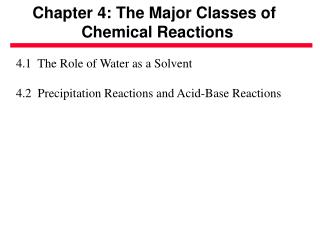Chapter 4: The Major Classes of Chemical Reactions

# Chapter 4: The Major Classes of Chemical Reactions - PowerPoint PPT Presentation

Chapter 4: The Major Classes of Chemical Reactions. 4.1 The Role of Water as a Solvent 4.2 Precipitation Reactions and Acid-Base Reactions. The Role of Water as a Solvent: The Solubility of Ionic Compounds.I am the owner, or an agent authorized to act on behalf of the owner, of the copyrighted work described.
Download Presentation## Chapter 4: The Major Classes of Chemical Reactions

An Image/Link below is provided (as is) to download presentation

Download Policy: Content on the Website is provided to you AS IS for your information and personal use and may not be sold / licensed / shared on other websites without getting consent from its author.While downloading, if for some reason you are not able to download a presentation, the publisher may have deleted the file from their server.

- - - - - - - - - - - - - - - - - - - - - - - - - - E N D - - - - - - - - - - - - - - - - - - - - - - - - - -
Presentation Transcript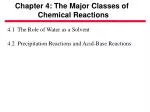Chapter 4: The Major Classes of

Chemical Reactions

4.1 The Role of Water as a Solvent

4.2 Precipitation Reactions and Acid-Base Reactions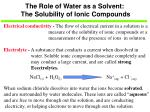Electrical conductivity - The flow of electrical current in a solution is a

measure of the solubility of ionic compounds or a

measurement of the presence of ions in solution.

Electrolyte - A substance that conducts a current when dissolved in

water. Soluble ionic compound dissociate completely and

may conduct a large current, and are called strong

Eeectrolytes.

NaCl(s) + H2O(l) Na+(aq) + Cl -(aq)

When sodium chloride dissolves into water the ions become solvated,

and are surrounded by water molecules. These ions are called “aqueous”

and are free to move through out the solution, and are conducting

electricity, or helping electrons to move through out the solution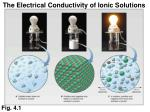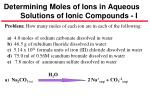Determining Moles of Ions in Aqueous

Solutions of Ionic Compounds - I

Problem: How many moles of each ion are in each of the following:

a) 4.0 moles of sodium carbonate dissolved in water

b) 46.5 g of rubidium fluoride dissolved in water

c) 5.14 x 1021 formula units of iron (III) chloride dissolved in water

d) 75.0 ml of 0.56M scandium bromide dissolved in water

e) 7.8 moles of ammonium sulfate dissolved in water

a) Na2CO3 (s) 2 Na+(aq) + CO3-2(aq)

H2O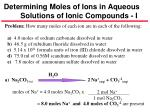Determining Moles of Ions in Aqueous

Solutions of Ionic Compounds - I

Problem: How many moles of each ion are in each of the following:

a) 4.0 moles of sodium carbonate dissolved in water

b) 46.5 g of rubidium fluoride dissolved in water

c) 5.14 x 1021 formula units of iron (III) chloride dissolved in water

d) 75.0 ml of 0.56M scandium bromide dissolved in water

e) 7.8 moles of ammonium sulfate dissolved in water

a) Na2CO3 (s) 2 Na+(aq) + CO3-2(aq)

moles of Na+ = 4.0 moles Na2CO3 x

= 8.0 moles Na+ and 4.0 moles of CO3-2 are present

H2O

2 mol Na+

1 mol Na2CO3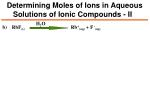Determining Moles of Ions in Aqueous

Solutions of Ionic Compounds - II

H2O

b) RbF(s) Rb+(aq) + F -(aq)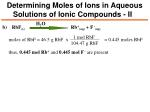Determining Moles of Ions in Aqueous

Solutions of Ionic Compounds - II

H2O

b) RbF(s) Rb+(aq) + F -(aq)

1 mol RbF

104.47 g RbF

moles of RbF = 46.5 g RbF x = 0.445 moles RbF

thus, 0.445 mol Rb+and 0.445 mol F -are present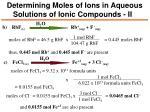Determining Moles of Ions in Aqueous

Solutions of Ionic Compounds - II

H2O

b) RbF(s) Rb+(aq) + F -(aq)

1 mol RbF

104.47 g RbF

moles of RbF = 46.5 g RbF x = 0.445 moles RbF

thus, 0.445 mol Rb+and 0.445 mol F -are present

H2O

c) FeCl3 (s) Fe+3(aq) + 3 Cl -(aq)

moles of FeCl3 = 9.32 x 1021 formula units

1 mol FeCl3

6.022 x 1023 formula units FeCl3

x

= 0.0155 mol FeCl3

3 mol Cl -

1 mol FeCl3

moles of Cl - = 0.0155 mol FeCl3 x = 0.0465 mol Cl -

and 0.0155 mol Fe+3 are also present.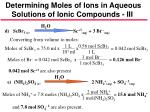Determining Moles of Ions in Aqueous

Solutions of Ionic Compounds - III

H2O

d) ScBr3 (s) Sc+3(aq) + 3 Br -(aq)

Converting from volume to moles:

1 L

103 ml

0.56 mol ScBr3

1 L

Moles of ScBr3 = 75.0 ml x x = 0.042 mol ScBr3

3 mol Br -

1 mol ScBr3

Moles of Br - = 0.042 mol ScBr3 x = 0.126 mol Br -

0.042 mol Sc+3 are also present

H2O

e) (NH4)2SO4 (s) 2 NH4+(aq) + SO4- 2(aq)

2 mol NH4+

1 mol(NH4)2SO4

Moles of NH4+ = 7.8 moles (NH4)2SO4 x = 15.6 mol NH4+

and 7.8 mol SO4- 2are also present.Determining Moles of Ions in Aqueous

Solutions of Ionic Compounds - III

H2O

d) ScBr3 (s) Sc+3(aq) + 3 Br -(aq)

Converting from volume to moles:

1 L

103 ml

0.56 mol ScBr3

1 L

Moles of ScBr3 = 75.0 ml x x = 0.042 mol ScBr3

3 mol Br -

1 mol ScBr3

Moles of Br - = 0.042 mol ScBr3 x = 0.126 mol Br -

0.042 mol Sc+3 are also present

H2O

e) (NH4)2SO4 (s) 2 NH4+(aq) + SO4- 2(aq)

2 mol NH4+

1 mol(NH4)2SO4

Moles of NH4+ = 7.8 moles (NH4)2SO4 x = 15.6 mol NH4+

and 7.8 mol SO4- 2are also present.The Solubility of Ionic Compounds in Water

The solubility of ionic compounds in water depends upon the relative

strengths of the electrostatic forces between ions in the ionic compound

and the attractive forces between the ions and water molecules in the

solvent. There is a tremendous range in the solubility of ionic

compounds in water! The solubility of so called “insoluble” compounds

may be several orders of magnitude less than ones that are called

“soluble” in water, for example:

Solubility of NaCl in water at 20oC = 365 g/L

Solubility of MgCl2 in water at 20oC = 542.5 g/L

Solubility of AlCl3 in water at 20oC = 699 g/L

Solubility of PbCl2 in water at 20oC = 9.9 g/L

Solubility of AgCl in water at 20oC = 0.009 g/L

Solubility of CuCl in water at 20oC = 0.0062 g/LThe Solubility of Covalent Compounds in Water

The covalent compounds that are very soluble in water are the ones

with -OH group in them and are called “polar” and can have strong

polar (electrostatic)interactions with water. Examples are compound

such as table sugar, sucrose (C12H22O11); beverage alcohol, ethanol

(C2H5-OH); and ethylene glycol (C2H6O2) in antifreeze.

H

Methanol = Methyl Alcohol

H

C

O H

H

Other covalent compounds that do not contain a polar center, or the

-OH group are considered “nonpolar” , and have little or no

interactions with water molecules. Examples are the hydrocarbons in

gasoline and oil. This leads to the obvious problems in oil spills, where

the oil will not mix with the water and forms a layer on the surface!

Octane = C8H18 and / or Benzene = C6H6Acids - A Group of Covalent Molecules Which Lose Hydrogen Ions to Water Molecules in Solution

When gaseous hydrogen iodide dissolves in water, the attraction of the

oxygen atom of the water molecule for the hydrogen atom in HI is

greater that the attraction of the of the iodide ion for the hydrogen atom,

and it is lost to the water molecule to form an hydronium ion and an

iodide ion in solution. We can write the hydrogen atom in solution as

either H+(aq) or as H3O+(aq) they mean the same thing in solution. The

presence of a hydrogen atom that is easily lost in solution is an “Acid”

and is called an “acidic” solution. The water (H2O) could also be written

above the arrow indicating that the solvent was water in which the HI

was dissolved.

HI(g) + H2O(L) H+(aq) + I -(aq)

HI(g) + H2O(L) H3O+(aq) + I -(aq)

H2O

HI(g) H+(aq) + I -(aq)Strong Acids and the Molarity of H+ Ions in

Aqueous Solutions of Acids

Problem: In aqueous solutions, each molecule of sulfuric acid will

loose two protons to yield two hydronium ions, and one sulfate ion.

What is the molarity of the sulfate and hydronium ions in a solution

prepared by dissolving 155g of concentrate sulfuric acid into sufficient

water to produce 2.30 Liters of acid solution?

Plan: Determine the number of moles of sulfuric acid, divide the moles

by the volume to get the molarity of the acid and the sulfate ion. The

hydronium ions concentration will be twice the acid molarity.

Solution: Two moles of H+ are released for every mole of acid:

H2SO4 (l) + 2 H2O(l) 2 H3O+(aq) + SO4- 2(aq)Strong Acids and the Molarity of H+ Ions in

Aqueous Solutions of Acids

Problem: In aqueous solutions, each molecule of sulfuric acid will

loose two protons to yield two hydronium ions, and one sulfate ion.

What is the molarity of the sulfate and hydronium ions in a solution

prepared by dissolving 155g of concentrate sulfuric acid into sufficient

water to produce 2.30 Liters of acid solution?

Plan: Determine the number of moles of sulfuric acid, divide the moles

by the volume to get the molarity of the acid and the sulfate ion. The

hydronium ions concentration will be twice the acid molarity.

Solution: Two moles of H+ are released for every mole of acid:

H2SO4 (l) + 2 H2O(l) 2 H3O+(aq) + SO4- 2(aq)

1 mole H2SO4

98.09 g H2SO4

Moles H2SO4 = = 1.58 moles H2SO4

155 g H2SO4

xStrong Acids and the Molarity of H+ Ions in

Aqueous Solutions of Acids

Problem: In aqueous solutions, each molecule of sulfuric acid will

loose two protons to yield two hydronium ions, and one sulfate ion.

What is the molarity of the sulfate and hydronium ions in a solution

prepared by dissolving 155g of concentrate sulfuric acid into sufficient

water to produce 2.30 Liters of acid solution?

Plan: Determine the number of moles of sulfuric acid, divide the moles

by the volume to get the molarity of the acid and the sulfate ion. The

hydronium ions concentration will be twice the acid molarity.

Solution: Two moles of H+ are released for every mole of acid:

H2SO4 (l) + 2 H2O(l) 2 H3O+(aq) + SO4- 2(aq)

1 mole H2SO4

98.09 g H2SO4

Moles H2SO4 = = 1.58 moles H2SO4

155 g H2SO4

x

1.58 mol SO4-2

2.30 l solution

Molarity of SO4- 2 = = 0.687 Molar in SO4- 2Strong Acids and the Molarity of H+ Ions in

Aqueous Solutions of Acids

Problem: In aqueous solutions, each molecule of sulfuric acid will

loose two protons to yield two hydronium ions, and one sulfate ion.

What is the molarity of the sulfate and hydronium ions in a solution

prepared by dissolving 155g of concentrate sulfuric acid into sufficient

water to produce 2.30 Liters of acid solution?

Plan: Determine the number of moles of sulfuric acid, divide the moles

by the volume to get the molarity of the acid and the sulfate ion. The

hydronium ions concentration will be twice the acid molarity.

Solution: Two moles of H+ are released for every mole of acid:

H2SO4 (l) + 2 H2O(l) 2 H3O+(aq) + SO4- 2(aq)

1 mole H2SO4

98.09 g H2SO4

Moles H2SO4 = = 1.58 moles H2SO4

155 g H2SO4

x

1.58 mol SO4-2

2.30 l solution

Molarity of SO4- 2 = = 0.687 Molar in SO4- 2

Molarity of H+ = 2 x 1.58 mol H+ / 2.30 liters = 1.38 Molar in H+Precipitation Reactions: A Solid Product is Formed

When ever two aqueous solutions are mixed, there is the possibility of

forming an insoluble compound. Let us look at some examples to see

how we can predict the result of adding two different solutions together.

Pb(NO3) (aq) + NaI(aq) Pb+2(aq) + 2 NO3-(aq) + Na+(aq) + I-(aq)

When we add these two solutions together, the ions can combine in the

way they came into the solution, or they can exchange partners. In this

case we could have lead nitrate and sodium iodide, or lead iodide and

sodium nitrate formed, to determine which will happen we must look at

the solubility table (p.141) to determine what could form. The table

indicates that lead iodide will be insoluble, so a precipitate will form.

Pb(NO3)2 (aq) + 2 NaI(aq) PbI2 (s)+ 2 NaNO3 (aq)Precipitation Reactions: Will a Precipitate Form?

If we add a solution containing potassium chloride to a solution

containing ammonium nitrate, will we get a precipitate?

KCl(aq) + NH4NO3 (aq) = K+(aq) + Cl-(aq) + NH4+(aq) + NO3-(aq)

By exchanging cations and anions we see that we could have potassium

chloride and ammonium nitrate, or potassium nitrate and ammonium

chloride. In looking at the solubility table it shows all possible

products as soluble, so there is no net reaction!

KCl(aq) + NH4NO3 (aq) = No Reaction!

If we mix a solution of sodium sulfate with a solution of barium nitrate,

will we get a precipitate? From the solubility table it shows that barium

sulfate is insoluble, therefore we will get a precipitate!

Na2SO4 (aq) + Ba(NO3)2 (aq) BaSO4 (s) + 2 NaNO3 (aq)Solubility Rules for Ionic Compounds in Water

Soluble Ionic Compounds Insoluble Ionic Compounds

1) All common compounds of

Group 1A(I) ions (Na+,K+, etc.)

and ammonium(NH4+) are soluble.

2) All common nitrates (NO3-),

acetates (CH3COO-), and most

perchlorates (ClO4-) are soluble.

3) All common chlorides (Cl-),

bromides (Br -), and iodides (I-) are

soluble, except those of Ag+, Pb2+,

Cu2+, and Hg22+.

4) All common sulfates (SO42-) are

soluble, except those of Ca+2, Sr2+,

Ba2+, and Pb2+.

1) All common metal hydroxides

are insoluble, except those of

Group 1A (1) and the larger

members of Group 2A(2)

(beginning with Ca2+).

2) All common carbonates (CO32-)

and phosphates (PO43-) are

insoluble, except those of

Group 1A(1) and NH4+.

3) All common sulfides are

insoluble, except those of

Group 1A(1), Group 2A(2),

and NH4+.Predicting Whether a Precipitation Reaction

Occurs; Writing Equations:

a) Calcium Nitrate and Sodium Sulfate solutions are added together.

Molecular Equation

Total Ionic Equation

Net Ionic Equation

-

b) Ammonium Sulfate and Magnesium Chloride are added together.Predicting Whether a Precipitation Reaction

Occurs; Writing Equations:

a) Calcium Nitrate and Sodium Sulfate solutions are added together.

Molecular Equation

Ca(NO3)2 (aq) + Na2SO4 (aq) CaSO4 (s) + NaNO3 (aq)

Total Ionic Equation

Net Ionic Equation

b) Ammonium Sulfate and Magnesium Chloride are added together.Predicting Whether a Precipitation Reaction

Occurs; Writing Equations:

a) Calcium Nitrate and Sodium Sulfate solutions are added together.

Molecular Equation

Ca(NO3)2 (aq) + Na2SO4 (aq) CaSO4 (s) + NaNO3 (aq)

Total Ionic Equation

Ca2+(aq)+2 NO3-(aq) + 2 Na+(aq)+ SO4-2(aq) CaSO4 (s) + 2 Na+(aq+) 2 NO3-(aq)

Net Ionic Equation

b) Ammonium Sulfate and Magnesium Chloride are added together.Predicting Whether a Precipitation Reaction

Occurs; Writing Equations:

a) Calcium Nitrate and Sodium Sulfate solutions are added together.

Molecular Equation

Ca(NO3)2 (aq) + Na2SO4 (aq) CaSO4 (s) + NaNO3 (aq)

Total Ionic Equation

Ca2+(aq)+2 NO3-(aq) + 2 Na+(aq)+ SO4-2(aq) CaSO4 (s) + 2 Na+(aq+) 2 NO3-(aq)

Net Ionic Equation

Ca2+(aq) + SO4-2(aq) CaSO4 (s)

Spectator Ions are Na+ and NO3-

b) Ammonium Sulfate and Magnesium Chloride are added together.Predicting Whether a Precipitation Reaction

Occurs; Writing Equations:

a) Calcium Nitrate and Sodium Sulfate solutions are added together.

Molecular Equation

Ca(NO3)2 (aq) + Na2SO4 (aq) CaSO4 (s) + NaNO3 (aq)

Total Ionic Equation

Ca2+(aq)+2 NO3-(aq) + 2 Na+(aq)+ SO4-2(aq) CaSO4 (s) + 2 Na+(aq+) 2 NO3-(aq)

Net Ionic Equation

Ca2+(aq) + SO-4(aq) CaSO4 (s)

Spectator Ions are Na+ and NO3-

b) Ammonium Sulfate and Magnesium Chloride are added together.

In exchanging ions, no precipitates will be formed, so there will be no

chemical reactions occurring! All ions are spectator ions!Acid - Base Reactions: Neutralization Rxns.

An Acid is a substance that produces H+ (H3O+) ions when dissolved

in water.

A Base is a substance that produces OH - ions when dissolved in water.

Acids and bases are electrolytes, and their strength is categorized in

terms of their degree of dissociation in water to make hydronium or

hydroxide ions. Strong acids and bases dissociate completely, and are

strong electrolytes. Weak acids and bases dissociate weakly and are

weak electrolytes.

The generalized reaction between an Acid and a Base is:

HX(aq) + MOH(aq) MX(aq) + H2O(L)

Acid + Base = Salt + WaterSelected Acids and Bases

Acids Bases

Strong Strong

Hydrochloric acid, HCl Sodium hydroxide, NaOH

Hydrobromic acid, HBr Potassium hydroxide, KOH

Hydriodoic acid, HI Calcium hydroxide, Ca(OH)2

Nitric acid, HNO3 Strontium hydroxide, Sr(OH)2

Sulfuric acid, H2SO4 Barium hydroxide, Ba(OH)2

Perchloric acid, HClO4

Weak Weak

Hydrofluoric acid, HF Ammonia, NH3

Phosphoric acid, H3PO4

Acidic acid, CH3COOH

(or HC2H3O2)

Table 4.2Writing Balanced Equations forNeutralization Reactions - I

Problem:Write balanced chemical reactions (molecular, total ionic, and

net ionic) for the following chemical reactions:

a) calcium hydroxide(aq) and hydroiodic acid(aq)

b) lithium hydroxide(aq) and nitric acid(aq)

c) barium hydroxide(aq) and sulfuric acid(aq)

Plan: These are all strong acids and bases, therefore they will make

water and the corresponding salts.

Solution:Writing Balanced Equations forNeutralization Reactions - I

Problem:Write balanced chemical reactions (molecular, total ionic, and

net ionic) for the following chemical reactions:

a) calcium hydroxide(aq) and hydroiodic acid(aq)

b) lithium hydroxide(aq) and nitric acid(aq)

c) barium hydroxide(aq) and sulfuric acid(aq)

Plan: These are all strong acids and bases, therefore they will make

water and the corresponding salts.

Solution:

a) Ca(OH)2 (aq) + 2HI(aq) CaI2 (aq) + 2H2O(l)Writing Balanced Equations forNeutralization Reactions - I

Problem:Write balanced chemical reactions (molecular, total ionic, and

net ionic) for the following chemical reactions:

a) calcium hydroxide(aq) and hydroiodic acid(aq)

b) lithium hydroxide(aq) and nitric acid(aq)

c) barium hydroxide(aq) and sulfuric acid(aq)

Plan: These are all strong acids and bases, therefore they will make

water and the corresponding salts.

Solution:

a) Ca(OH)2 (aq) + 2HI(aq) CaI2 (aq) + 2H2O(l)

Ca2+(aq) + 2 OH -(aq) + 2 H+(aq) + 2 I -(aq)

Ca2+(aq) + 2 I -(aq) + 2 H2O(l)Writing Balanced Equations forNeutralization Reactions - I

Problem:Write balanced chemical reactions (molecular, total ionic, and

net ionic) for the following chemical reactions:

a) calcium hydroxide(aq) and hydroiodic acid(aq)

b) lithium hydroxide(aq) and nitric acid(aq)

c) barium hydroxide(aq) and sulfuric acid(aq)

Plan: These are all strong acids and bases, therefore they will make

water and the corresponding salts.

Solution:

a) Ca(OH)2 (aq) + 2HI(aq) CaI2 (aq) + 2H2O(l)

Ca2+(aq) + 2 OH -(aq) + 2 H+(aq) + 2 I -(aq)

Ca2+(aq) + 2 I -(aq) + 2 H2O(l)

2 OH -(aq) + 2 H+(aq) 2 H2O(l)Writing Balanced Equations for

Neutralization Reactions - II

b) LiOH(aq) + HNO3 (aq) LiNO3 (aq) + H2O(l)Writing Balanced Equations for

Neutralization Reactions - II

b) LiOH(aq) + HNO3 (aq) LiNO3 (aq) + H2O(l)

Li+(aq) + OH -(aq) + H+(aq) + NO3-(aq)

Li+(aq) + NO3-(aq) + H2O(l)

OH -(aq) + H+(aq)H2O(l)Writing Balanced Equations for

Neutralization Reactions - II

b) LiOH(aq) + HNO3 (aq) LiNO3 (aq) + H2O(l)

Li+(aq) + OH -(aq) + H+(aq) + NO3-(aq)

Li+(aq) + NO3-(aq) + H2O(l)

OH -(aq) + H+(aq)H2O(l)

c) Ba(OH)2 (aq) + H2SO4 (aq) BaSO4 (s) + 2H2O(l)Writing Balanced Equations for

Neutralization Reactions - II

b) LiOH(aq) + HNO3 (aq) LiNO3 (aq) + H2O(l)

Li+(aq) + OH -(aq) + H+(aq) + NO3-(aq)

Li+(aq) + NO3-(aq) + H2O(l)

OH -(aq) + H+(aq)H2O(l)

c) Ba(OH)2 (aq) + H2SO4 (aq) BaSO4 (s) + 2H2O(l)

Ba2+(aq) + 2OH -(aq) + 2 H+(aq) + SO42-(aq) BaSO4 (s) + 2 H2O(l)

Ba2+(aq) + 2 OH -(aq) + 2 H+(aq) + SO42-(aq) BaSO4 (s) + 2 H2O(l)Volume (L) of base (difference in

M (mol/L) of base

Moles of base

molar ratio

Moles of acid

volume (L) of acid

M (mol/L) of acidProblem: A titration is performed between sodium hydroxide and

potassium hydrogenphthalate (KHP) to standardize the base solution,

by placing 50.00 mg of solid potassium hydrogenphthalate in a flask

with a few drops of an indicator. A buret is filled with the base, and the

initial buret reading is 0.55 ml; at the end of the titration the buret

reading is 33.87 ml. What is the concentration of the base?

Plan: Use the molar mass of KHP (204.2 g/mol) to calculate the number

of moles of the acid, from the balanced chemical equation, the reaction

is equal molar, so we know the moles of base, and from the difference

in the buret readings, we can calculate the molarity of the base.

Solution:

HKC8H4O4 (aq) + OH -(aq) KC8H4O4-(aq) + H2O(aq)O

C

O

K+

O

H

C

O

Potassium Hydrogenphthalate KHC8H4O4

O

C

K+

O

O

C

H+

OFinding the Concentration of Base from an Acid - Base Titration - II

50.00 mg KHP

204.2 g KHP

1 mol KHP

1.00 g

1000 mg

moles KHP = x = 0.00024486 mol KHP

Volume of base = Final buret reading - Initial buret reading

= 33.87 ml - 0.55 ml = 33.32 ml of base

one mole of acid = one mole of base; therefore 0.00024486 moles of

acid will yield 0.00024486 moles of base in a volume of 33.32 ml.

0.00024486 moles

0.03332 L

molarity of base = = 0.07348679 moles per liter

molarity of base = 0.07349 MAcid + Base Salt + H2O

Fig. 4.9An Acid-Base Reaction That Formsa Gaseous Product

The reaction of acid with carbonates or bicarbonates will

produce carbon dioxide gas that is released from solution as a gas in the form of bubbles that leave the solution.

Fig. 4.10Types of Chemical Reactions - I

I) Combination reactions that are redox reactions

a) A metal and a nonmetal form an Ionic compound

b) Two nonmetals form a covalent compound

c) Combination of an element and a compound

II) Combination reactions that are not redox reactions

a) A metal oxide and a nonmetal form an ionic compound with

a polyatomic anion

b) Metal oxides and water form bases

c) Nonmetal oxides and water form acids

III) Decomposition reactions

a) Thermal decomposition

i) Many ionic compounds with oxoanions form a metal oxide

and a gaseous nonmetal

ii) Many metal oxides, chlorates, and perchlorates release

oxygen

b) Electrolytic decompositionTypes of Chemical Reactions - II

IV) Displacement Reactions

a) Single-Displacement Reactions - Activity Series of the Metals

i) A metal displaces hydrogen from water or an acid

ii) A metal displaces another metal ion from solution

iii) A halogen displaces a halide ion from solution

b) Double-Displacement Reactions -

i) In precipitation reactions: A precipitate forms

ii) In acid-base reactions: Acid + Base form a salt & water

V) Combustion Reactions - All are Redox Processes

a) Combustion of an element with oxygen to form oxides

b) Combustion of hydrocarbons to yield water & carbon dioxide

Reactants

ProductsTwo Nonmetals Combine to Form a Binary

Covalent Compound - Redox Rxns

Halogens of nonmetals

P4 (s) + 6 F2 (g) 4 PF3 (g)

I2 (s) + 5 F2 (g) 2IF5 (l)

S8 (s) +4 Br2 (l) 4 S2Br2 (l)

N2 (g) + 3 Cl2 (g) 2 NCl3 (g)

Nitrides and sulfides

P4 (s) +10 N2 (g) 4 P3N5 (s)

S8 (s) +2 N2 (g) 2 S4N2 (s)

8 P4 (s) + 5 S8 (s) 8 P4S5 (s)Other Elements Combine with Oxygen to Form Oxides

Metals combining with xygen

4 Na(s) + O2 (g) 2 Na2O(s)

2 Ca(s) + O2 (g) 2 CaO(s)

4 Al(s) +3 O2 (g) 2 Al2O3 (s)

Ti(s) + O2 (g) TiO2 (s)

Nonmetals with oxygen

N2 (g) + O2 (g) 2 NO (g)

P4 (s) + 5 O2 (g) P4O10 (s)

S8 (s) +8 O2 (g) 8 SO2 (g)

2 F2 (g) + O2 (g) 2 OF2 (g)Nonmetal Oxides & Halides React with

Additional Oxygen & Halogens to Form

“Higher” Oxides and Halides

1)Nonmetal oxides with oxygen:

2 NO(g) + O2 (g) 2 NO2 (g)

P4O6 (s) +2 O2 (g) P4O10 (s)

2 CO(g) + O2 (g) 2 CO2 (g)

2) Nonmetal halides with halogens:

ClF(g) + F2 (g) ClF3 (g)

BrF3 (g) +F2 (g) BrF5 (l)

IF3 (g) + F2 (g) IF5 (l)

IF5 (l) + F2 (g) IF7 (l)Combination of a Compound and an Element:

Nonmetal Oxides & Halides React with Additional Oxygen

and Halogens to Form “Higher” Oxides and Halides

1)Nonmetal oxides with oxygen:

2 NO(g) + O2 (g) 2 NO2 (g)

P4O6 (s) +2 O2 (g) P4O10 (s)

2 CO(g) + O2 (g) 2 CO2 (g)

2) Nonmetal halides with halogens:

ClF(g) + F2 (g) ClF3 (g)

PF3 (g) +F2 (g) PF5 (l)

IF3 (g) + F2 (g) IF5 (l)

IF5 (l) + F2 (g) IF7 (l)Combination of Two Compounds - I

1) Metal oxide with a nonmetal oxide to form an ionic compound

with a polyatomic anion.

Na2O(s) + CO2 (g) Na2CO3 (s)

K2O(s) + SO2 (g) K2SO3 (s)

CaO(s) + SO3 (g) CaSO4 (s)

2) Metal oxides react with water to form hydroxides

Na2O(s) + H2O(l) 2 NaOH(aq)

BaO(s) + H2O(l) Ba(OH)2 (s)

2 Sc2O3 (s) + 6 H2O(l) 4 Sc(OH)3 (s)

FeO(s) + H2O(l) Fe(OH)2 (s)Combination of Two Compounds - II

3) Nonmetal oxides react with water to form acids.

CO2 (g) + H2O(l) H2CO3 (aq)

SO2 (g) + H2O(l) H2SO3 (aq)

3 NO2 (g) + H2O(l) 2 HNO3 (aq) + NO(g)

4) Hydrates result from the reaction of anhydrous (without water)

compounds with water.

.

CuSO4 (s) + 5 H2O(l) CuSO4 5H2O (s)

.

MgSO4 (s) + 7 H2O(l) MgSO4 7H2O(s)

.

Na2CO3 (s) + 10 H2O(l) Na2CO3 10H2O(s)

5) Addition reactions with carbon compounds.

C2H4 (g) + Cl2 (g) C2H4Cl2 (g)

Ethylene + Chlorine dichloroethaneThermal Decomposition Reactions - I

Carbonates Oxides and carbon dioxide

Na2CO3 (s) Na2O(s) + CO2 (g)

MgCO3 (s) MgO(s) + CO2 (g)

Sulfites Oxides and sulfur dioxide

MgSO3 (s) MgO(s) + SO2 (g)

K2SO3 (s) K2O(s) + SO2 (g)

Metal oxides, chlorates, and perchlorates Oxygen

2 Na2O(s) 4 Na(s) + O2 (g)

KClO4 (s) KCl(s) + 2 O2 (g)Thermal Decomposition Reactions - II

Hydroxides, hydrates, and some oxoacids Water

Ca(OH)2 (s) CaO(s) + H2O(g)

Na2CO3 10H2O (s) Na2CO3 (s) + 10 H2O(g)

H2SO3 (l) SO2 (g) + H2O(g)

2 Fe(OH)3 (s) Fe2O3 (s) + 3 H2O(g)

MgSO4 7H2O(s) MgSO4 (s) + 7 H2O(g)

H2CO3 (aq) CO2 (g) + H2O(g)

2 NaOH(s) Na2O(s) + H2O(g)Metals Displace Hydrogen from Water

Metals that will displace ydrogen from cold water:

2 Cs(s) + 2 H2O H2 (g) + 2 CsOH(aq)

Ba(s) +2 H2O(l) H2 (g) + Ba(OH)2 (aq)

2 Na(s) + 2 H2O(l) H2 (g) + 2 NaOH(aq)

Metals that will displace hydrogen from steam:

Mg(s) + 2 H2O(g) H2 (g) + Mg(OH)2 (s)

2 Cr(s) + 6 H2O(g) 3 H2 (g) +2 Cr(OH)3 (s)

Zn(s) + 2 H2O(g) H2 (g) + Zn(OH)2 (s)Metals Displace Hydrogen from Acids

Reactions of metals above hydrogen in the activity series

Mg(s) + 2 HCl(aq) MgCl2 (aq) + H2 (g)

Zn(s) + H2SO4 (aq) ZnSO4 (aq) + H2 (g)

2 Al(s) + 6 HCl(aq) 2 AlCl3 (aq) + 3 H2 (g)

Cd(s) + 2 HBr(aq) CdBr2 (aq) + H2 (g)

Sn(s) + 2 H2SO4 (aq) Sn(SO4)2 (aq) + 2 H2 (g)

Reactions of metals below hydrogen in the activity series

Cu(s) + HCl(aq) No Reaction!

Au(s) + H2SO4 (aq) No Reaction!Single-Displacement Reactions -

Metals Replace Metal Ions from Solution

Ba(s) + Co(NO3)2 (aq) Co(s) + Ba(NO3)2 (aq)

Cd(s) + AgNO3 (aq) 2 Ag(s) + Cd(NO3)2 (aq)

Mg(s) + Pb(NO3)2 (aq) Pb(s) + Mg(NO3)2 (aq)

Al(s) + Ba(NO3)2 (aq) No Reaction

4 Cr(s) + 3 PtCl4 (aq) 3 Pt(s) + 4 CrCl3 (aq)

Ca(s) + Hg(NO3)2 (aq) Hg(l) + Ca(NO3)2 (aq)

2 Li(s) + Na2SO4 (aq) + H2O(l) 2 NaOH(aq) + H2 (g) + Li2SO4 (aq)Double-Displacement Reactions

1) Precipitation reactions - an insoluble product is formed:

Pb(NO3)2 (aq) + 2 NaI(aq) PbI2 (s) + 2 NaNO3 (aq)

Ba(NO3)2 (aq) + Na2SO4 (aq) BaSO4 (s) + 2 NaNO3 (aq)

2) Acid-base neutralization reactions - water is formed:

HCl(aq) + NaOH(aq) NaCl(aq) + H2O(l)

H2SO4 (aq) + Ca(OH)2 (s) CaSO4 (s) + 2 H2O(l)

3) A carbonate or sulfite reacts with acid to form a gas:

Na2CO3 (aq) + 2 HBr(aq) 2 NaBr(aq) + H2O(l) + CO2 (g)

K2SO3 (aq) + 2 HI(aq) 2 KI(aq) + H2O(l) + SO2 (g)Combustion Reactions: Redox Reactions

Elements combining with oxygen to form oxides:

2 Al(s) + 3 O2 (g) 2 Al2O3 (s)

S8 (s) + 8 O2 (g) 8 SO2 (g)

C(s) + O2 (g) CO2 (g)

Compounds combining with oxygen to form oxides

2 Fe2S3 (s) + 9 O2 (g) 2 Fe2O3 (s) + 6 SO2 (g)

2 Ca3N2 (s) + 7 O2 (g) 6 CaO(s) + 4 NO2 (g)

Hydrocarbons combining with oxygen to form CO2 and H2O

CH4 (g) + 2 O2 (g) CO2 (g) + 2 H2O(g)

2 C4H10 + 18 O2 (g) 8 CO2 (g) + 10 H2O(g)Identifying the Type of Chemical Reaction - I

Problem: Classify the following as combination, decomposition, or

displacement reactions, identify the underlying chemical process as

precipitation, acid-base, or redox, and write a balanced molecular

equation for each. For redox, identify the oxidizing and reducing agents.

a) Barium chloride(aq) and ammonium sulfate(aq)

b) Manganese metal and tin IV chloride (aq)

c) Strontium hydroxide and bromic acid

d) Cobalt metal and nitrogen gas to yield cobalt II nitride

e) Sodium peroxide to give sodium oxide and oxygen gas

Plan: Identify the reaction type, and identify the products and equation.

Solution:

a) Displacement (metathesis): two substances form two others, one of

which is a precipitate.

BaCl2 (aq) + (NH4)2SO4 (aq) BaSO4 (s) + 2 NH4Cl(aq)Identifying the Type of Chemical Reaction -II

b) Displacement reaction(single): This “redox” reaction occurs when

the more active manganese displaces the less reactive tin.

2 Mn(s) + SnCl4 (aq) 2 MnCl2 (aq) + Sn(s)

Mn is the reducing agent, and SnCl4 is the oxidizing agent.

c) Displacement reaction(metathesis): Two substances form two others.

This is an acid-base reaction. The base Sr(OH)2 reacts with twice as

much bromic acid to give water and the salt, strontium bromate

dissolved in water.

Sr(OH)2 (aq) + 2 HBrO3 (aq) Sr(BrO3)2 (aq) + 2 H2O(L)Identifying the Type of Chemical Reaction -III

d) Combination Reaction: This redox reaction occurs when cobalt

metal is heated in nitrogen gas and forms the solid compound

cobalt II nitride.

3 Co(s) + N2 (g) Co3N2 (s)

Nitrogen is the oxidizing agent, and is reduced; cobalt is the

reducing agent, and is oxidized.

e) Decomposition reaction: One substance forms two substances. This

is a redox reaction that forms oxygen gas.

2 Na2O2 (s) 2 Na2O(s) + O2 (g)

Sodium peroxide is the reducing and oxidizing agent, as oxygen

is both oxidized, and reduced.